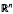Home

# Maximum principles for parabolic equations

## Abstract

Let u(x, t) be a smooth function in the domain Q = Ω × (0, L), Ω inn, let Du be the spatial gradient of u(x, t) and let ∇u = (Du, u1). If u(x, t) satisfies the parabolic equation F(u, Du, D2u) = ut, we define w(x, t) by g(w) = │∇u−1G(∇u) (g is positive and decreasing, G is concave and homogeneous of degree one) and we prove that w(x, t) attains its maximum value on the parabolic boundary of Q. If u(x, t) satisfies the equation Δu + 2h(q2) uiujuij = ut(q2 = │Du│2, 1 + 2q2h(q2) > 0) we prove that qf (u) takes its maximum value on the parabolic boundary of Q provided f satisfies a suitable condition. If u(x, t) satisfies the parabolic equation aij (Du)uij − b(x, t, u, Du) = ut (b is concave with respect to (x, t, u)) we define C(x, y, t, τ) = u(z, θ) − αu(x, t) − βu(y, τ) (0 < α, 0 < β, α + β = 1, z αx +y, θ = αt + βτ) and we prove that if C(x, y, t, r) ≤0 when x, y, z ∈ Ω2 and one of t, τ = 0, and when t, τ ∈ (0, L], and one of x, y, z, ∈ ∂Ω, then it is C(x, y, t, τ) ≤0 everywhere.

• # Send article to Kindle

Note you can select to send to either the @free.kindle.com or @kindle.com variations. ‘@free.kindle.com’ emails are free but can only be sent to your device when it is connected to wi-fi. ‘@kindle.com’ emails can be delivered even when you are not connected to wi-fi, but note that service fees apply.

Find out more about the Kindle Personal Document Service.

Maximum principles for parabolic equations
Available formats
×

# Send article to Dropbox

To send this article to your Dropbox account, please select one or more formats and confirm that you agree to abide by our usage policies. If this is the first time you use this feature, you will be asked to authorise Cambridge Core to connect with your <service> account. Find out more about sending content to Dropbox.

Maximum principles for parabolic equations
Available formats
×

# Send article to Google Drive

To send this article to your Google Drive account, please select one or more formats and confirm that you agree to abide by our usage policies. If this is the first time you use this feature, you will be asked to authorise Cambridge Core to connect with your <service> account. Find out more about sending content to Google Drive.

Maximum principles for parabolic equations
Available formats
×

## References

Hide All
MathJax
MathJax is a JavaScript display engine for mathematics. For more information see http://www.mathjax.org.

# Maximum principles for parabolic equations

## Metrics

### Full text viewsFull text views reflects the number of PDF downloads, PDFs sent to Google Drive, Dropbox and Kindle and HTML full text views.

Total number of HTML views: 0
Total number of PDF views: 0 *Loading metrics...

### Abstract viewsAbstract views reflect the number of visits to the article landing page.

Total abstract views: 0 *Loading metrics...

* Views captured on Cambridge Core between <date>. This data will be updated every 24 hours.

Usage data cannot currently be displayed.